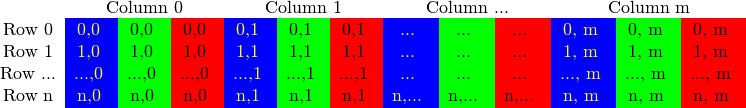# opencv2-新特性及Mat

相对于cpp接口的源码来说，它就有很多好处了，比如不需要像之前需要用 c 语言来模拟 cpp的面向对象那样，能够更加的模块化，而且对于编程人员来说，新增的cpp接口中增加的智能指针类，可以大大减少编程人员所需的自己申请 自己释放内存的问题。（其实 个人更加喜欢cpp，自然各种好话往上堆啊，哈哈）。

#include <opencv2/core/core.hpp>
#include <opencv2/imgproc/imgproc.hpp>
#include <opencv2/highgui/highgui.hpp>

3.1Mat类的初始化

1、考虑到OpenCV中会对大图像进行操作，如果一两幅也就算了，可是总有经常拷贝图像和视频帧的时候，这时候如果频繁的拷贝数据，那么就很慢了，而且大部分的情况下程序员的想法也是想对同一幅图像数据进行操作，所以OpenCV将这个想法作为默认想法，如果真想在复制一副图像的同样的数据，那么就显式的调用函数。在OpenCV中一般会有（opencv1中有图像或者矩阵头，和图像或者矩阵数据部分 两个部分），这里也是一样，只不过将默认拷贝矩阵头和指向数据的指针进行复制（有点像cpp语言特性中的引用）。

Mat A, C;                                 // 只创建信息头部分

Mat B(A);                                 // 使用拷贝构造函数

C = A;                                    // 赋值运算符
Mat D (A, Rect(10, 10, 100, 100) ); // using a rectangle
Mat E = A(Range:all(), Range(1,3)); // using row and column boundaries

Mat F = A.clone();
Mat G;
A.copyTo(G);

2、上面的是从图像中读取数据，这才有的矩阵，但是如果想平地而起的创建咋办？那就得使用Mat类的其他构造函数了。这里不介绍多，尽量往简单通用的地方靠，等使用opencv多了，那就可以往深的地方看看：

Mat M(2,2, CV_8UC3, Scalar(0,0,255));
或者  Mat R = Mat(3, 2, CV_8UC3); randu(R, Scalar::all(0), Scalar::all(255));
int sz = {2,2,2};      Mat L(3,sz, CV_8UC(1), Scalar::all(0));
上面第一行是建立个二维矩阵：参数为行、列、元素类型、每个元素的初始化值。CV_8UC3，8就是8 bit，U是无符号，C是通道，在OpenCV中是通过宏来定义的，具体的可以参见<types_c.h>的584-625行。ps：对于二维矩阵有个特例就是可以直接cout<<M<<endl; 因为内部实现了对2维矩阵的直接输出。在官方文档的具体输出部分也是只介绍了二维矩阵在不同格式下的显示（不过因为暂时只玩过matlab和cpp，那些python啥的没接触，也就不介绍了 http://www.opencv.org.cn/opencvdoc/2.3.2/html/doc/tutorials/core/mat%20-%20the%20basic%20image%20container/mat%20-%20the%20basic%20image%20container.html#matthebasicimagecontainer 这里的格式化打印部分，有兴趣的看看，个人觉得这个是在黑框显示的，只是给程序员看内部数据的，所以觉得有一个用得着就够了，介绍多了，容易晕）。

上面第二行是先建立矩阵大小，然后不指定初始化值，通过使用randu（）函数来随机指定，其中需要有随机数的上下界。

上面第三行是多维矩阵的建立，先是一个多维矩阵每个维度上的大小，CV_8UC(1) 就是指定多少通道，因为默认只有1 2 3 4 ，所以其他维度就得自己指定（这里也算是多此一举，因为可以使用CV_8UC1 代替的）。

3、Matlab类型的初始化

Mat M;    M.create(4,4, CV_8UC(2));
    cout << "M = "<< endl << " "  << M << endl << endl;
    Mat E = Mat::eye(4, 4, CV_64F);

Mat O = Mat::ones(2, 2, CV_32F);

Mat Z = Mat::zeros(3,3, CV_8UC1);

上面第一行是一个创建函数，为了开辟一个指定大小的数据空间，其中的值由205初始化，通道数影响的是每一行的长度，比如这里实际开辟的是4×8的矩阵大小。然后随之直接输出结果，记得这里只有二维矩阵才有这待遇使用cout<<;

接下来的三行就是因为Matlab所属公司和OpenCV小组合作的结果，这样才有这么便捷的初始化（个人：这里的理解在cpp语法上应该是先创建一个临时类对象，然后进行eye等的初始化和数据空间的创建，然后在将临时类对象赋值给矩阵E、 O、 Z，具体的可以看《C++程序设计语言 》或者《C++primer》中的有关章节）。关于CV_64F的定义可以查看<types_c.h>中569-576行

3.2 Mat类对象的访问方法

这里要说下在OpenCV中的数据表示方法，如下图所示，是每一行中长度也就是矩阵的列数是由 （点×通道数）  决定，而行数是不变的，而且是BGR排序，不是RGB排序，这个排序的顺序，完全是最初的大牛制定的时候卖萌罢了。即：

Mat I = imread("your picture",CV_LOAD_IMAGE_COLOR);
int nCols = I.cols*I.channels();
int  nRows =I .rows;按照上面的代码接着写

 if (I.isContinuous())
{
nCols *= nRows;
nRows = 1;
}

    uchar* p;
for(size_t i = 0; i < nRows; ++i)
{
p = I.ptr<uchar>(i);
for (size_t j = 0; j < nCols; ++j)
{
}
}

template<typename _Tp> inline _Tp* Mat::ptr(int y)
{
CV_DbgAssert( y == 0 || (data && dims >= 1 && (unsigned)y < (unsigned)size.p) );
return (_Tp*)(data + step.p*y);
}

方法2：迭代器的方式访问

 const int channels = I.channels();
switch(channels)
{
case 1:
{
MatIterator_<uchar> it, end;
for( it = I.begin<uchar>(), end = I.end<uchar>(); it != end; ++it)
break;
}
case 3:
{
MatIterator_<Vec3b> it, end;
for( it = I.begin<Vec3b>(), end = I.end<Vec3b>(); it != end; ++it)
{
}
}
}


uchar：typedef unsigned char uchar;

Vec3b：typedef  Vec<uchar, 3> Vec3b;

Vec：template<typename _Tp, int cn> class Vec;

switch(channels)
{
case 1:
{
for( size_t i = 0; i < nRows; ++i)
for( size_t j = 0; j < nCols; ++j )
break;
}
case 3:
{
Mat_<Vec3b> _I = I;                  for( size_t i = 0; i < nRows; ++i){
for( size_t j = 0; j < nCols; ++j ) {                                     _I(i,j) = /*your operation*/;    
                    _I(i,j) = /*your operation*/;
_I(i,j) = /*your operation*/;  
                     }
                     I = _I;
                    break;
               }
       }

posted @ 2014-12-16 15:57  仙守  阅读(2108)  评论(0编辑  收藏  举报